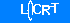Welcom to Shenzhen kedida Electronics Co., Ltd.Technology News

# Calculation Method pH

2016-04-19 15:42:12

PH value is calculated as follows: where [H] refers to a solution of hydrogen ion activity (sometimes written as [H3O], hydrated hydrogen ion activity), the unit is mol / liter.

In dilute solutions, the hydrogen ion activity is approximately equal to the concentration of hydrogen ions, the hydrogen ion concentration can be approximately calculated. At standard temperature and pressure, pH = 7 aqueous solution (such as: water) is neutral, it is because the water at standard temperature and pressure naturally ionized hydrogen ions and hydroxide ion concentration of the product (water ions product constant) is always 1 × 10 ^ -14, and the concentration of the two ions is 1 × 10 ^ -7mol / L.

Description pH less than 7 OH concentration greater than the concentration of H, so the solution is strongly acidic, and the pH is greater than 7 is less than the described concentration of H OH concentration, it is strong alkaline solution. So the pH value is smaller, the stronger the acid solution; pH greater the alkaline solution also stronger.

In the non-aqueous solution or non-standard conditions of temperature and pressure, pH = 7 may not represent the solution was neutral, which need to calculate the ionization constant of the solvent under such conditions to determine neutral pH value. Under such 373K (100 ℃) temperature, pH = 6 neutral solution.

Online messageMessageOnline Customer Service